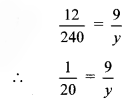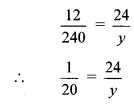# Maharashtra Board 8th Class Maths Practice Set 7.1 Solutions Chapter 7 Variation

Balbharti Maharashtra State Board Class 8 Maths Solutions covers the Practice Set 7.1 8th Std Maths Answers Solutions Chapter 7 Variation.

## Practice Set 7.1 8th Std Maths Answers Chapter 7 Variation

Question 1.
Write the following statements using the symbol of variation.

1. Circumference (c) of a circle is directly proportional to its radius (r).
2. Consumption of petrol (l) in a car and distance traveled by that car (d) are in direct variation.

Solution:

1. c ∝ r
2. l ∝ d

Question 2.
Complete the following table considering that the cost of apples and their number are in direct variation.

 Number of apples (x) 1 4 __ 12 __ Cost of apples (y) 8 32 56 __ 160

Solution:
The cost of apples (y) and their number (x) are in direct variation.
∴y ∝ x
∴y = kx …(i)
where k is the constant of variation

i. When, x = 1, y = 8
∴ Substituting, x = 1 and y = 8 in (i), we get y = kx
∴ 8 = k × 1
∴ k = 8
Substituting k = 8 in (i), we get
y = kx
∴ y = 8x …(ii)
This the equation of variation

ii. When,y = 56, x = ?
∴ Substituting y = 56 in (ii), we get
y = 8x
∴ 56 = 8x
∴ x = $$\frac { 56 }{ 8 }$$
∴ x = 7

iii. When, x = 12, y = ?
∴ Substituting x = 12 in (ii), we get
y = 8x
∴ y = 8 × 12
∴ y = 96

iv. When, y = 160, x = ?
∴ Substituting y = 160 in (ii), we get
y = 8x
∴ 160 = 8x
∴ x = $$\frac { 160 }{ 8 }$$
∴ x = 20

 Number of apples (x) 1 4 7 12 20 Cost of apples (y) 8 32 56 96 160

Question 3.
If m ∝ n and when m = 154, n = 7. Find the value of m, when n = 14.
Solution:
Given that,
m ∝ n
∴ m = kn …(i)
where k is constant of variation.
When m = 154, n = 7
∴ Substituting m = 154 and n = 7 in (i), we get
m = kn
∴ 154 = k × 7
∴ $$k=\frac { 154 }{ 7 }$$
∴ k = 22
Substituting k = 22 in (i), we get
m = kn
∴ m = 22n …(ii)
This is the equation of variation.
When n = 14, m = ?
∴ Substituting n = 14 in (ii), we get
m = 22n
∴ m = 22 × 14
∴ m = 308

Question 4.
If n varies directly as m, complete the following table.

 m 3 5 6.5 __ 1.25 n 12 20 __ 28 __

Solution:
Given, n varies directly as m
∴ n ∝ m
∴ n = km …(i)
where, k is the constant of variation

i. When m = 3, n = 12
∴ Substituting m = 3 and n = 12 in (i), we get
n = km
∴ 12 = k × 3
∴ $$k=\frac { 12 }{ 3 }$$
∴ k = 4
Substituting, k = 4 in (i), we get
n = km
∴ n = 4m …(ii)
This is the equation of variation.

ii. When m = 6.5, n = ?
∴ Substituting, m = 6.5 in (ii), we get
n = 4m
∴ n = 4 × 6.5
∴ n = 26

iii. When n = 28, m = ?
∴ Substituting, n = 28 in (ii), we get
n = 4m
∴ 28 = 4m
∴ 28 = 4m
∴ $$m=\frac { 28 }{ 4 }$$
∴ m = 7

iv. When m = 1.25, n = ?
∴ Substituting m = 1.25 in (ii), we get
n = 4m
∴ n = 4 × 1.25
∴ n = 5

 m 3 5 6.5 7 1.25 n 12 20 26 28 5

Question 5.
y varies directly as square root of x. When x = 16, y = 24. Find the constant of variation and equation of variation.
Solution:
Given, y varies directly as square root of x.
∴ y ∝ √4x
∴ y = k √x …(i)
where, k is the constant of variation.
When x = 16 ,y = 24.
∴ Substituting, x = 16 and y = 24 in (i), we get
y = k√x
∴24 = k√16
∴24 = 4k
∴ $$k=\frac { 24 }{ 4 }$$
∴ k = 6
Substituting k = 6 in (i), we get
y = k√x
∴ y = 6√x
This is the equation of variation
∴ The constant of variation is 6 and the equation of variation is y = 6√x .

Question 6.
The total remuneration paid to laborers, employed to harvest soybean is in direct variation with the number of laborers. If remuneration of 4 laborers is Rs 1000, find the remuneration of 17 laborers.
Solution:
Let, m represent total remuneration paid to laborers and n represent number of laborers employed to harvest soybean.
Since, the total remuneration paid to laborers, is in direct variation with the number of laborers.
∴ m ∝ n
∴ m = kn …(i)
where, k = constant of variation
Remuneration of 4 laborers is Rs 1000.
i. e., when n = 4, m = Rs 1000
∴ Substituting, n = 4 and m = 1000 in (i), we get m = kn
∴ 1000 = k × 4
∴ $$k=\frac { 1000 }{ 4 }$$
∴ k = 250
Substituting, k = 250 in (i), we get
m = kn
∴ m = 250 n …(ii)
This is the equation of variation
Now, we have to find remuneration of 17 laborers.
i. e., when n = 17, m = ?
∴ Substituting n = 17 in (ii), we get
m = 250 n
∴ m = 250 × 17
∴ m = 4250
∴ The remuneration of 17 laborers is Rs 4250.

Maharashtra Board Class 8 Maths Chapter 7 Variation Practice Set 7.1 Intext Questions and Activities

Question 1.
If the rate of notebooks is Rs 240 per dozen, what is the cost of 3 notebooks?
Also find the cost of 9 notebooks, 24 notebooks and 50 notebooks and complete the following table. (Textbook pg. no. 35)

 Number of notebooks (x) 12 3 9 24 50 1 Cost (In Rupees) (y) 240 __ __ __ __ 20

Solution:
As the number of notebooks increases their cost also increases.
∴ Number of notebooks and cost of notebooks are in direct proportion.

i.∴ y = 3 × 20
∴ y = 60

ii.∴ y = 9 × 20
∴ y = 180

iii.∴ y = 24 × 20
∴ y = 480

iv.∴ y = 50 × 20
∴ y = 1000

 Number of notebooks (x) 12 3 9 24 50 1 Cost (In Rupees) (y) 240 60 180 480 1000 20
Scroll to Top